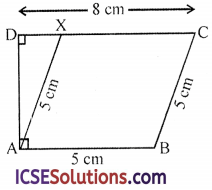Students can track their progress and improvement through regular use of S Chand Class 9 Maths Solutions ICSE Chapter 16 Area of Plane Figures Ex 16(C).

## S Chand Class 9 ICSE Maths Solutions Chapter 16 Area of Plane Figures Ex 16(C)

Question 1.
Find the area of a parallelogram whose base and height are as given below.

 Base 8 cm 2.8 cm 12 cm 6.5 cm Height 3 cm 5 cm 8.7 cm 4.8 cm

Solution:
(i) Base (b) = 8 cm
Height (h) = 3 cm
∴ Area = bh = 8 × 3 = 24 cm2

(ii) Base (b) = 2.8 cm
Height (h) = 5 cm
∴ Area = bh = 2.8 × 5 = 14 cm2

(iii) Base (b) = 12 mm
Height (h) = 8.7 mm
Area = bh = 12 × 8.7 = 104.4 mm2

(iv) Base (b) = 6.5 m
Height (h) = 4.8 m
Area = bh = 6.5 × 4.8 = 31.20 m2

Question 2.
The area of a parallelogram is 1$$\frac { 1 }{ 2 }$$ ares. Its base is 20 m. Find its height (1 are = 100 m2).
Solution:
Area of a parallelogram = 1$$\frac { 1 }{ 2 }$$ ares
= 1.5 × 100 = 150 m2
Base (b) = 20 m
Height (h) = $$\frac{\text { Area }}{\text { Base }}$$ = $$\frac { 150 }{ 20 }$$ = 7.5 mQuestion 3.
In a parallelogram ABCD, AB = 8 cm, BC = 5 cm, perp. from A to DC = 3 cm. Find the length of the perp. drawn from B to AD.
Solution:
In ||gm ABCD,
AB = 8 cm, BC = 5 cm
and perp. AL on DC = 3 cm
Let perp. BM on DA = x
Now area of the ||gm ABCD
= Base × Height
= DC × AL = 8 × 3 = 24 cm2
If base is AD = BC = 5 cm
Then height BM = $$\frac{\text { Area }}{\text { Base }}$$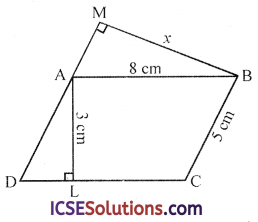Question 4.
A parallelogram has sides 34 cm and 20 cm. One of its diagonals is 42 cm. Calculate its area.
Solution:
In ||gm ABCD,
AB = 34 cm, AD = 20 cm
and diagonal BD = 42 cm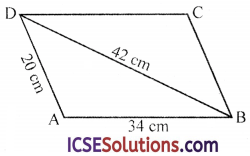∵ Each diagonals of a ||gm bisect it into two triangles of equal area
∴ Area of ||gm ABCD = 2 area △ABD Now in △ABD,Question 5.
ABCD is a parallelogram with side AB = 12 cm. Its diagonal AC and BD are of lengths 20 cm and 16 cm respectively. Find the area of ||gm ABCD.
Solution:
Side AB of ||gm ABCD = 12 cm
Diagonal AC = 20 cm
and diagonal BD = 16 cm∵ Diagonals of a ||gm bisect each other
∴ AO = OC = $$\frac { 20 }{ 2 }$$ = 10 cm
and BO = OD = $$\frac { 16 }{ 2 }$$ = 8 cm
Now area of △AOB with sides 12 cm, 10 cm, 8 cm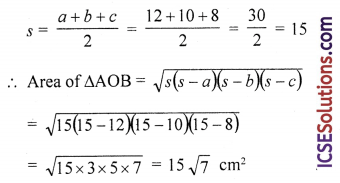∴ Diagonals of a ||gm form 4 equal triangles
∴ Area of ||gm ABCD = 4 × area △AOB
= 4 × 15 √7 = 60√7 cm2

Question 6.
What is the area of a rhombus which has diagonals of 8 cm and 10 cm.
Solution:
Diagonals of a rhombus ABCD are AC = 8 cm, BD= 10 cm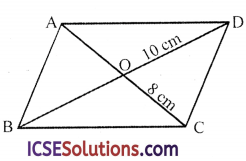∴ Area = $$\frac{\text { Product of diagonals }}{2}$$ = $$\frac{\mathrm{AC} \times \mathrm{BD}}{2}$$
= $$\frac{8 \times 10}{2}$$ = 40 cm2Question 7.
The area of a rhombus is 98 cm2. If one of its diagonals is 14 cm, what is the length of the other diagonal ?
Solution:
Area of rhombus = 98 cm2
and length of one diagonal = 14 cm
Second diagonal = $$\frac{\text { Area } \times 2}{1 \text { st diagonal }}$$ = $$\frac{98 \times 2}{14}$$ = 14 cm2

Question 8.
PQRS is a rhombus.
(i) If it is given that PQ = 3 cm, calculate the perimeter of PQRS.
(ii) If the height of the rhombus is 2.5 cm, calculate its area.
(iii) The diagonals of a rhombus are 8 cm and 6 cm respectively. Find its perimeter.
Solution:
(i) In rhombus PQRS,
Side PQ = 3 cm
∴ Perimeter = 4 × side = 4 × 3 = 12 cm

(ii) Height of rhombus PQRS = 2.5 cm
∴ Area = base + height = 3 × 2.5 = 7.5 cm2

(iii) Diagonals are 8 cm and 6 cm of rhombus PQRS
∵ Diagonals of a rhombus bisect each other at right angles∴ In right △POQ,
PO = 4 cm, QO = 3 cm
∴ PQ2 = PO2 + QO2 (Pythagoras Theorem)
(4)2 + (3)2 = 16 + 9 = 25
= (5)2
∴ Side PQ = 5 cm
and perimeter = 4 × side = 4 × 5 = 20 cm

Question 9.
The sides of a rhombus are 5 cm each and one diagonal is 8 cm, calculate,
(i) The length of the other diagonal and
(ii) The area of the rhombus.
Solution:
Each side of rhombus = 5 cm
and one diagonal = 8 cm
∵ Diagonals of a rhombus bisect each other at right angle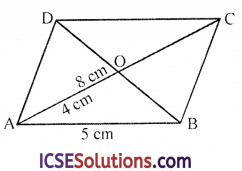(i) ∴ In right △AOB,
AO = $$\frac { 1 }{ 2 }$$ AC = $$\frac { 8 }{ 2 }$$ = 4 cm and BO = $$\frac { 1 }{ 2 }$$ BD
= AB2 = AO2 + BO2
(5)2 = (4)2 + BO2
⇒ 25 = 16 + BO2
⇒ BO2 = 25 – 16 = 9 = (3)2
∴ BO = 3 cm
∴ BD = 2 × BO = 2 × 3 = 6 cm

(ii) Area = $$\frac{\text { Product of diagonals }}{2}$$
= $$\frac{8 \times 6}{2}$$ = 24 cm2

Question 10.
In the figure, ABCX is a rhombus of side 5 cm. Angles BAD and ADC are right angles. If DC = 8 cm, calculate the area of ABCX.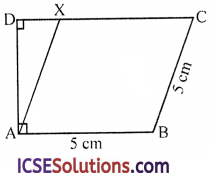Solution:
In the figure, ABCX is a rhombus with each side = 5 cm.